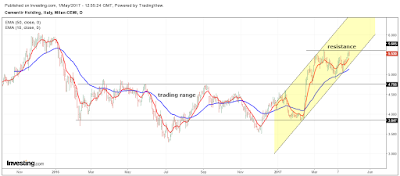## Monday, May 1, 2017

### Cement industry : Cementir Holding S.p.A. and the value map.

Here we have a classic value map that relates the market multiple P/S (price to sales) to its intrinsic profitability (EBIT/sales or EBIT margin or ROS) and then the mentioned multiple to the expected growth, from the year 2017 to the year 2019 (data source estimates : www.4-traders.com).

The expected growth is the CAGR, aka cumulative average growth rate.
The formula is the following :

[(expected revenues fiscal year 2019/revenues fiscal year 2016)]^(1/3)-1

It is expressed as a percentage.

The meaning of the relationships (P/S-ROS and P/S-CAGR) is that higher the multiple (P/S), higher the margin (ROS) and higher the multiple (P/S), higher the expected growth (CAGR) and viceversa.
Obviously, the market generally rewards the firms with higher profitability and with higher growth, with a major market price.
The relationships can be shown as a value map, particularly through a regression line.
We have two equations :

1) P/S = a + b*ROS

P/S = y ; ROS = x

2) P/S = a + b*CAGR

P/S = y ; CAGR = x

In both cases, the intercept (a) was removed for the reason of the low statistical relevance.
The RSQ is high (0.9070 and 0.8488), which means the strenght of the model.
Graphically, we can note that the relations are clear, substantially : higher the market multiple, higher the profitability and the expected growth.

The stocks above the regression line (dashed line) are overvalued, the stocks below the regression line are undervalued. In this way, for Cementir Holding, we get a "value gap" that is equal to :

[P/S (effective)-P/S(calculated from the market model)] : [P/S(calculated from
the market model)]

The P/S (effective) is 0.72.
The P/S (calculated from the market model) is equal to (9.10%*12.54).

There are also some "outsiders" (Vulcan Materials, Eagle Materials). The profitability is very high compared to the peers. Indeed, we have a multiple P/S of about 4X.

Likewise, for the relationship P/S-CAGR : see the following chart.

The discount ("value gap") is much greater, 71.94% vs 36.91%. The "growth adjusted market multiple" is preferable to the "multiple adjusted for the profitability".

If we remove the two outsiders from the sample, the statistical models are stronger : RSQ is higher, the relations are more even significative. However, the discounts are lower : 10.36% vs 36.91% and 57.16% vs 71.94%.

Finally, Cementir Holding is cheaper than the peers. Secondly, in my opinion, the stock is much more attractive in the area 3.80-4.80 : we could benefit more so from the discount prices and from a possible graphic retracement (see the chart), event if the current prices and expectations are interesting.Chart from Investing.com